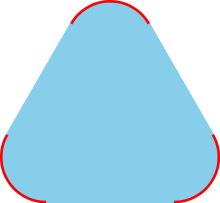# Krein–Milman theoremGiven a convex shape K (light blue) and its set of extreme points B (red), the convex hull of B is K.

In the mathematical theory of functional analysis, the Krein–Milman theorem is a proposition about convex sets in topological vector spaces. A particular case of this theorem, which can be easily visualized, states that given a convex polygon, one only needs the corners of the polygon to recover the polygon shape. The statement of the theorem is false if the polygon is not convex, as then there can be many ways of drawing a polygon having given points as corners.

Formally, letbe a locally convex topological vector space (assumed to be Hausdorff), and letbe a compact convex subset of. Then, the theorem states thatis the closed convex hull of its extreme points.

The closed convex hull above is defined as the intersection of all closed convex subsets ofthat containThis turns out to be the same as the closure of the convex hull in the topological vector space. One direction in the theorem is easy; the main burden is to show that there are 'enough' extreme points.

The original statement proved by Mark Krein and David Milman was somewhat less general than this.

Hermann Minkowski had already proved that ifis finite-dimensional thenequals the convex hull of the set of its extreme points. The Krein–Milman theorem generalizes this to arbitrary locally-convex, with a caveat: the closure may be needed.

## Relation to the axiom of choice

The axiom of choice, or some weaker version of it, is needed to prove this theorem in Zermelo–Fraenkel set theory. This theorem together with the Boolean prime ideal theorem, though, can prove the axiom of choice.

## Related results

Under the previous assumptions onifis a subset ofand the closed convex hull ofis all of, then every extreme point ofbelongs to the closure ofThis result is known as Milman's (partial) converse to the Krein–Milman theorem.

The Choquet–Bishop–de Leeuw theorem states that every point inis the barycenter of a probability measure supported on the set of extreme points of## More general settings

The assumption of local convexity for the ambient space is necessary, because James Roberts constructed a counter-example in 1977 for more general spaces.

The linearity is also needed, because the statement fails for weakly compact convex sets in CAT(0) spaces, as proved in 2016 by Nicolas Monod. However, Theo Buehler proved in 2006 that the Krein–Milman theorem does hold for metrically compact CAT(0) spaces. 

## References

• M. Krein, D. Milman (1940) On extreme points of regular convex sets, Studia Mathematica 9 133–138.
• Milman, D. (1947). Характеристика экстремальных точек регулярно-выпуклого множества [Characteristics of extremal points of regularly convex sets]. Doklady Akademii Nauk SSSR (in Russian). 57: 119–122.
• H. Minkowski. Geometrie der Zahlen. Teubner, Leipzig, 1910
• N. Monod (2016) "Extreme points in non-positive curvature", Studia Mathematica 234 265–270.
• N. K. Nikol'skij (Ed.). Functional Analysis I. Springer-Verlag, 1992
• J. Roberts (1977) A compact convex set with no extreme points, Studia Mathematica 60 255–266.
• H. L. Royden. Real Analysis. Prentice-Hall, Englewood Cliffs, New Jersey, 1988.